Open in App
Not now

# Class 9 NCERT Solutions- Chapter 13 Surface Areas And Volumes – Exercise 13.7

• Last Updated : 04 Mar, 2021

### (ii) radius 3.5 cm, height 12 cm

Solution:

Volume of cone (V) = (1/3) × πr2h

(i) Given values,

Radius of cone (r) = 6 cm

Height of cone (h) = 7 cm

V = (1/3) × (22/7) × 6 × 6 × 7             (using π=22/7)

V = 22 × 6 × 2

V = 264 cm3

(ii) Given values,

Radius of cone (r) = 3.5 cm

Height of cone (h) = 12 cm

V = (1/3) × (22/7) × 3.5 × 3.5 × 12           (using π=22/7)

V = 154 cm3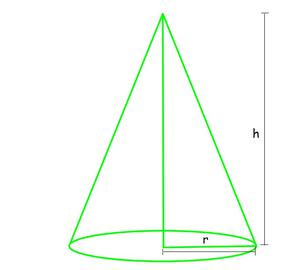### (ii) height 12 cm, slant height 13 cm

Solution:

Volume of cone (V) = (1/3) × πr2h

(i) Given values,

Radius of cone (r) = 7 cm

Slant height of cone (l) = 25 cm

h = √(l2 – r2)

h = √(252 – 72)

h = √576

h = 24 cm

V = (1/3) × (22/7) × 7 × 7 × 24                         (using π=22/7)

V = 22 × 7 × 8

V = 1232 cm3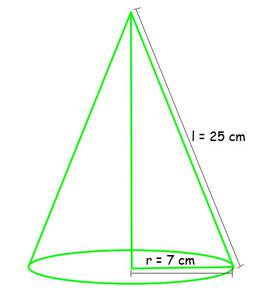(ii) Given values,

Height of cone (h) = 12 cm

Slant height of cone (l) = 13 cm

r = √(l2 – h2)

r = √(132 – 122)

r = √25

r = 5 cm

V = (1/3) × (22/7) × 5 × 5 × 12                            (using π=22/7)

V = 2200/7 cm3

V = 314.28 cm3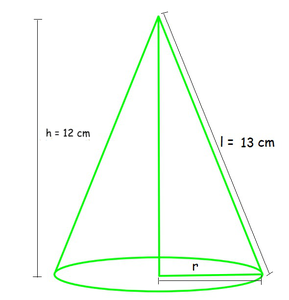### Question 3. The height of a cone is 15 cm. If its volume is 1570 cm3, find the radius of the base. (Use π = 3.14)

Solution:

Given values,

Height of cone (h) = 15 cm

Volume of cone (V) = 1570 cm3

V = (1/3) ×  πr2h

1570 = (1/3) × 3.14 × r2 × 15                         (using π=3.14)

r2 = 1570 × 3 / (3.14 × 15)

r2 = 100

r = √100

r = 10 cm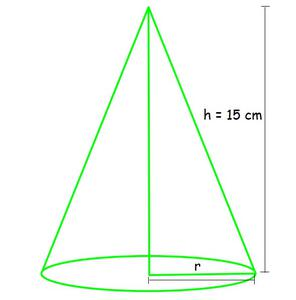### Question 4. If the volume of a right circular cone of height 9 cm is 48 π cm3, find the diameter of its base.

Solution:

Given values,

Height of cone (h) = 9 cm

Volume of cone (V) = 48π cm3

V = (1/3) × πr2h

48 × π = (1/3) × π × r2 × 9

r2 = 48 × 3 / 9          (canceling π from both sides)

r2 = 16

r = √16

r = 4 cm

Diameter = 2 times radius = 2 × r

= 2 × 4

= 8 cm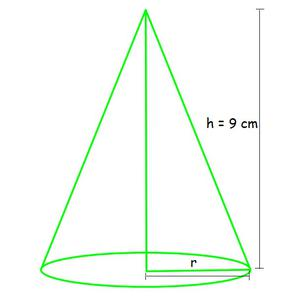### Question 5. A conical pit of top diameter 3.5 m is 12 m deep. What is its capacity in kiloliters?

Solution:

Given values,

Radius of cone (r) = 3.5/2 m

Height of cone (h) = 12 m

Volume of cone = (1/3) × πr2h

= (1/3) × (22/7) × (3.5/2) × (3.5/2) × 12                (taking π=22/7)

= (22 × 3.5 × 3.5 × 12) / (7 × 3 × 2 × 2)

= 38.5 m3

Capacity of conical pit in kilo liters:

1000 m3 = 1 liter

38.5 m3 = 1000 × 38.5 liters

= 38,500 liters

= 38.5 kilo liters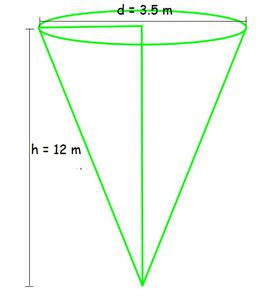### (iii) curved surface area of the cone

Solution:

Given values,

Radius of cone (r) = 28/2 = 14 cm

Volume of cone (V) = 9856 cm3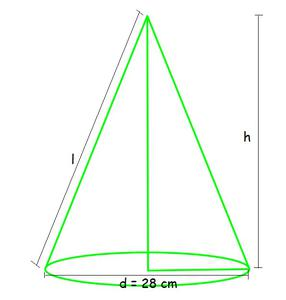(i) Volume of cone = (1/3) × πr2h

9856 = (1/3) × (22/7) × 14 × 14 × h                   (taking π=22/7)

h = (9856 × 3 × 7) / (22 × 14 × 14)

h = 48 cm

(ii) Let slant height = l

l2 = h2 + r2

l = √(h2 + r2)

l = √(482 + 142)

l = √(2304 + 196)

l = √2500

l = 50 cm

(iii) curved surface area of the cone = πrl

= π × 14 × 50 cm2

= 22/7 × 700                                   (taking π=22/7)

= 2,200 cm2

### Question 7. A right triangle ABC with sides 5 cm, 12 cm, and 13 cm is revolved about the side 12 cm. Find the volume of the solid so obtained.

Solution:

Here, after revolving the triangle about the side 12 cm, we get

Radius of cone (r) =5 cm

Height of cone (h) = 12 cm

Volume of cone = (1/3) × πr2h

= (1/3) × π × 5 × 5 × 12

= (12 × π × 5 × 5) / 3

V = 100π cm3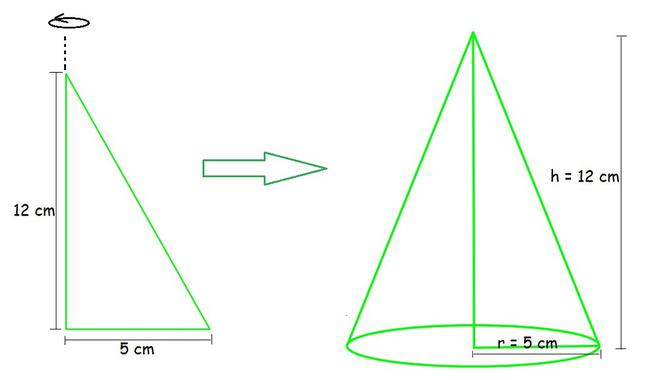### Question 8. If the triangle ABC in the Question 7 above is revolved about the side 5 cm, then find the volume of the solid so obtained. Find also the ratio of the volumes of the two solids obtained in Questions 7 and 8.

Solution:

Here, after revolving the triangle about the side 5 cm, we get

Radius of cone (r) =12 cm

Height of cone (h) = 5 cm

Volume of cone = (1/3) × πr2h

= (1/3) × π × 12 × 12 × 5

= (12 × π × 12 × 5) / 3

V = 240π cm3

Volume in Question 7 = 100π cm3

Ratio = (Volume in Question 8) / (Volume in Question 7)

= 240π/100π

= 12/5

Hence, the ratio obtained = 12 : 5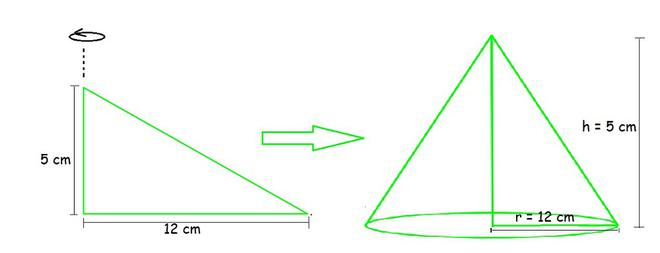### Question 9. A heap of wheat is in the form of a cone whose diameter is 10.5 m and height is 3 m. Find its volume. The heap is to be covered by canvas to protect it from rain. Find the area of the canvas required.

Solution:

Given values,

Radius of cone (r) = 10.5/2 = 105/20 m

Height of cone (h) = 3 m

Volume of cone = (1/3) × πr2h

= (1/3) × (22/7) × (105/2) × (105/2) × 3                (taking π=22/7)

= (22 × 105 × 105 × 3) / (3 × 20× 20× 7)

V = 86.625 m3

Area of the canvas = surface area of cone = πrl

Slant height (l) = √(h2 + r2)

l = √(32 + (10.5/2)2)

l = √(9+ (110.25/4))

l = √(146.25/4)

l = √36.56

l = 6.05 m (approx.)

Surface area of cone = π × (105/20) × 6.05 m2

= (22/7) × (635.25/20)                                         (taking π=22/7)

= 99.82 m2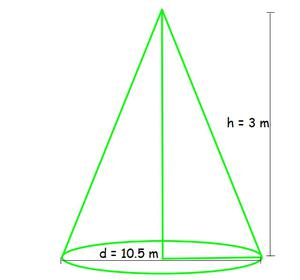My Personal Notes arrow_drop_up
Related Articles